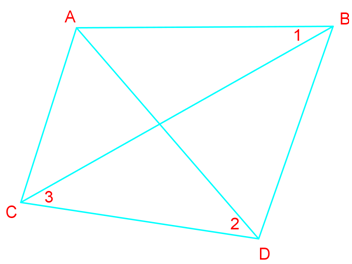# Parallel line proofs#### All You Need in One Place

Everything you need for better marks in primary, GCSE, and A-level classes.#### Learn with Confidence

We’ve mastered the UK’s national curriculum so you can study with confidence.#### Instant and Unlimited Help

0/1
##### Intros
###### Lessons
1. Relationships between lines and angles
0/10
##### Examples
###### Lessons
1. What is the value of x if $\overline {AB}$ $\parallel$ $\overline {CD}$ ?

1.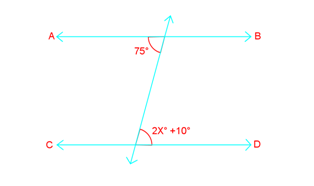2.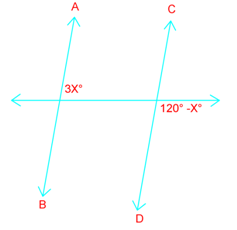3.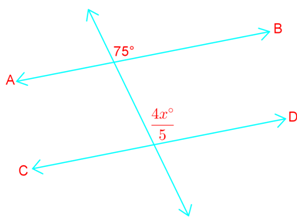2. Determine if enough information is given to prove $p \parallel$ $q$ State the postulates or theorems you use in your answers.

1.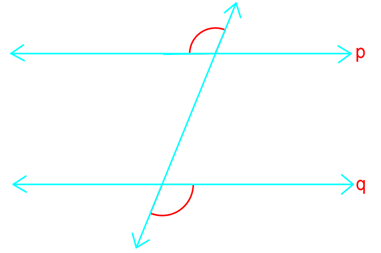2.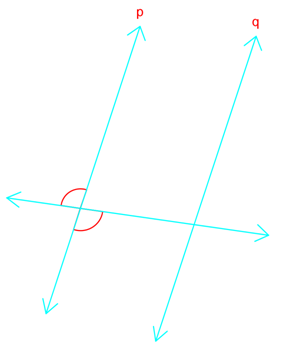3.4.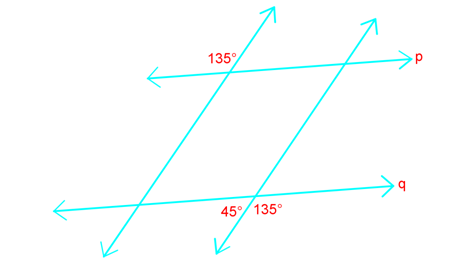3. Prove that if two lines are cut by a transversal so the alternate exterior angles are congruent, then the lines are parallel. (Prove the Alternate Exterior Angles converse)
1. Write a two-column proof.
1. Given: $m \parallel$ $n$, $\angle 1$ $\cong$$\angle 2$
Prove: $s \parallel$ $t$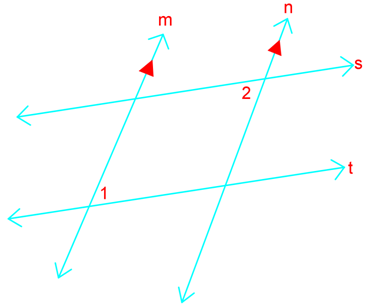2. Given: $\angle 1$ $\cong$$\angle 2$, $\angle 1$ $\cong$$\angle 3$
Prove: $\overline {AB}$ $\parallel$ $\overline {CD}$